About Qweas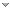RSS Feeds     BBS Forum Make Qweas.com My Home Page     Bookmark this page Register     Login     Help     Send Feedback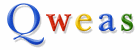All software Windows Mac Linux Mobile Games Screensavers Source Code Webware
New Software  |  Software CatalogsWin 98/Other/2000/XP/Vista, Mac OS X
Mathpad is an easy to use text editor for mathematics. You can mix together ordinary text and any mathematical expression. Ideal for math teachers to create quizzes, tests and handouts. Also, you can save the formatted text as an image. With most equation editors you choose a template with the mouse, type a few keystrokes and repeat the process. Mathpad doesn't work this way. Mainly, you just type characers at the keyboard.## ?Math for kids1

Win Other/98/XP
Educational math software for school or personal. 4 elementary math action covering math with numbers, fractions, decimals.## MathProf4.0

Win 98/Other/XP/2000/Server
MathProf is an easy to use mathematics program within approximately 180 subroutines. MathProf can display mathematical correlations in a very clear and simple way. The program covers the areas Analysis, Geometry, Algebra, Stochastics,Vector algebra.MathProf helps Junior High School students with problems in Geometry and Algebra. High School and College students, seeking to expand their knowledge into further reaching mathematical concepts find th## MathSuga16.3

Win XP/Vista/Vista x64/2000/98/Server
MathSuga features include evaluation of mathematical equations. Generation of graphs from equations. Drawing of two dimensional objects such as lines, circles, rectangles. Writing text, saving previously drawn objects to components.## JMathLib0.9.4

Win Other/98/2000/XP/Vista, Linux/Gnome/GPL/Open Source, U...
JMathLib is a clone of Matlab but written 100% in java. It can be extended very simply with m-files an java classes. It is intended to be a java version of programs such as MatLab, Octave, FreeMat and Scilab.## Math Worm1.0

Win Other/98/XP/2000
A puzzle where you delve into a mathematical maze for answers to simple arithmetic equations. You must navigate the underground maze using numbers as clues while digging a tunnel for your math worm to get home. Mark the tunnel by completing adjacent arit## MathCards1.0

Win Other/98/2000/XP
Math Cards helps students reinforce their math. Children and teenagers can play against the computer or a partner in this mathematical facts game. Reinforce whole numbers, integers, fractions, roots, percents, exponents, equations and roman numerals.## Math Logic4.0

Win 98/Other/2000/XP
Math Logic 4.0 is a math help & learning software for children that is an integral component of math lesson plans. Math Logic is a fun and straightforward computerized online math games method of learning and solving math problems for school students## Speed Math3.0

Win 98/Other/2000/XP
Speed Math 3.0 is a very easy and interesting Kids math games for learning Arithmetic. The fun math games software has been designed for ease of use, fast setup and interactivity.## Flash Math1.04

Pocket PC
Flash Math scales problems according to children's proficiency so that they are not frustrated by difficult problems or unchallenged by less intricate equations.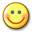## ZinsMath3.0

Win 98/Other/2000/XP## KidsMath1.02

Win 2000/XP
A cool easy to use calculator thats easy to use an does alot.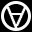## LiteralMath2.5

Win 98/2000/XP
Text editor with math notation and hypertext. Useful in high school / college.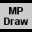Win 98/Other/2000/XP/Vista
Math drawing program for both two and three dimensional objects.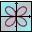## Math Studio2.1

Win Other/XP/2000
Math tool for high school teachers and students.## Math Flight2.3

Win Other/98/2000/XP/Server/Vista/Vista x64
Fly High while having fun learning basic mathematics with Math Flight!## AnalyticMath1.1.4

Win 98/Other/2000/XP/Server
Free math / graphing program - an excellent tool for function visualization.## Easy Math2.0

Palm
Exercises for Multiplication, Division, Subtraction, Addition, Fractions, etc.## QB - Math2.0

Win 98/Other/XP
Practice Multiplication, Addition, Subtraction and Division!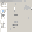## ChemMaths13.0

Win Other/2000/XP/Server/Vista/Vista x64
Software suitable for Engineering,Chemistry/Science, Professional/education.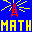## Internet Math1.0

Win Other/98/XP/2000
Uses the Internet to teach how to solve math word problems grades 1-8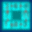## Mixed-Up Math1.0

Win Other/98/XP/2000
A math puzzle where equations are all mixed-up. Equations go in all directions.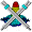## Math Mechanixs1.5.0.1

Win 2000/Server/Vista/Vista x64/XP
A scientific and engineering, math, statistics and graphing tool.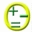## Math Flash4.6

Win XP/Vista/7 x32/Other
Flash card style addition and subtraction practice.## MathShapesPro2X2.2

Win 98/Other/2000/XP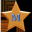## Math Stars Plus2010

Win 2000/XP/Server/Vista, Mac OS X
Practice math facts. Also includes 6 fun and challenging math games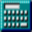## Math Calculator2.1.1

Win Other/XP/2000
Math calculator can calculate expression, derivative, root, extremum, integral.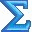## MathType for Mac6.0

Mac OS X
MathType for Macintosh is a powerful interactive equation editor.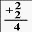## Math Flash Cards1

Win 98/2000/XP
Learn math the easy way. Practice with Math Flash Cards. Great for all ages.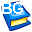## Mathematics Quiz2

Win 98/Other/2000/XP/Server
For students aged 7 - 9 in Primary 1 - 3. 1500 maths quizzes & problems sums